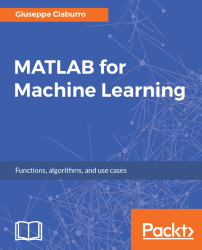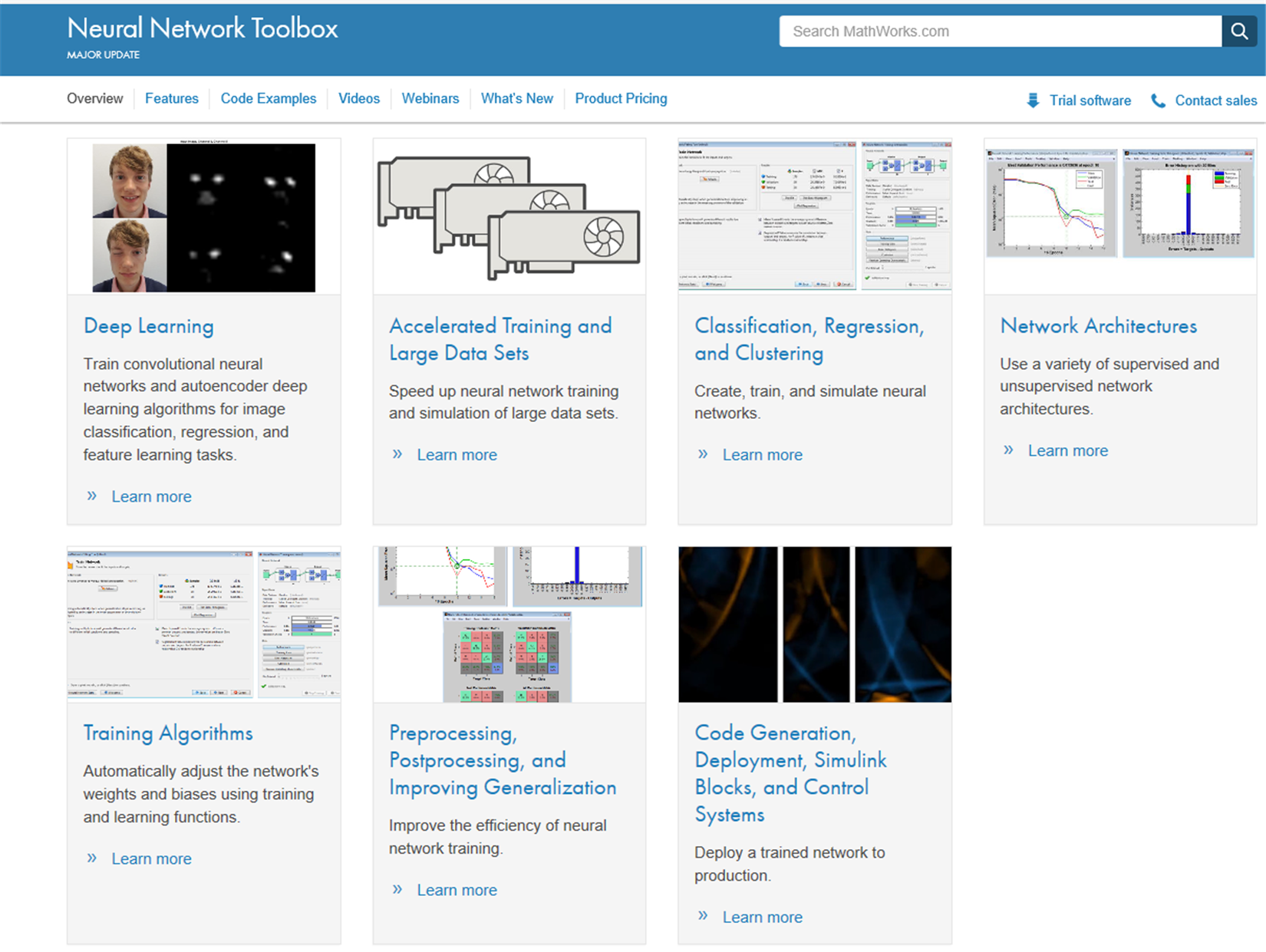•#### MATLAB for Machine Learning#### Overview of this book

MATLAB is the language of choice for many researchers and mathematics experts for machine learning. This book will help you build a foundation in machine learning using MATLAB for beginners. You’ll start by getting your system ready with t he MATLAB environment for machine learning and you’ll see how to easily interact with the Matlab workspace. We’ll then move on to data cleansing, mining and analyzing various data types in machine learning and you’ll see how to display data values on a plot. Next, you’ll get to know about the different types of regression techniques and how to apply them to your data using the MATLAB functions. You’ll understand the basic concepts of neural networks and perform data fitting, pattern recognition, and clustering analysis. Finally, you’ll explore feature selection and extraction techniques for dimensionality reduction for performance improvement. At the end of the book, you will learn to put it all together into real-world cases covering major machine learning algorithms and be comfortable in performing machine learning with MATLAB.
Title PageCreditsForewordwww.PacktPub.comCustomer FeedbackPrefaceFree Chapter
Getting Started with MATLAB Machine LearningImporting and Organizing Data in MATLABFrom Data to Knowledge DiscoveryFinding Relationships between Variables - Regression TechniquesPattern Recognition through Classification AlgorithmsIdentifying Groups of Data Using Clustering MethodsSimulation of Human Thinking - Artificial Neural NetworksImproving the Performance of the Machine Learning Model - Dimensionality ReductionMachine Learning in Practice## Neural Network Toolbox

ANNs are a computational model used in computer science, built on a large series of simple neural units, called artificial neurons, which draw inspiration from the behavior observed in the axons of a human brain. Each neural unit is connected with many others, and such link defines the activation status of the adjacent neural units. Every single neural unit performs calculations using the summation function. The models based on ANNs are self-learning and training, rather than explicitly programmed, which his is particularly suitable in cases where the solution function is hard to express in a traditional computer program.

The Neural Network Toolbox provides algorithms, pre-trained models, and apps to create, train, visualize, and simulate neural networks with one hidden layer (called shallow neural network) and neural networks with several hidden layers (called deep neural networks). Through the use of the tools offered, we can perform classification, regression, clustering, dimensionality reduction, time series forecasting, and dynamic system modeling and control.

Deep learning networks include convolutional neural networks (CNNs) and autoencoders for image classification, regression, and feature learning. For training sets of moderated sized, we can quickly apply deep learning by performing transfer learning with pre-trained deep networks. To make working on large amounts of data faster, we can use the Parallel Computing Toolbox (another MATLAB toolbox) to distribute computations and data across multicore processors and GPUs on the desktop, and we can scale up to clusters and clouds with MATLAB Distributed Computing Server.

Here is a descriptive list of the key features of this tool; you will find the main topics of the machine learning field here:

• Deep learning with CNNs (for classification and regression) and autoencoders (for feature learning)
• Transfer learning with pre-trained CNNs models and models from the Caffe model zoo
• Training and inference with CPUs or multi-GPUs on desktops, clusters, and clouds
• Unsupervised learning algorithms, including self-organizing maps and competitive layers

In the following screenshot, the product capabilities of the `Neural Networks Toolbox` are shown, extracted from the MathWorks site:Figure 1.21: Product capabilities of the Neural Networks Toolbox

• Supervised learning algorithms, including multilayer, radial basis, Learning Vector Quantization (LVQ), time-delay, nonlinear autoregressive (NARX), and Recurrent Neural Network (RNNs)
• Apps for data fitting, pattern recognition, and clustering
• Preprocessing, postprocessing, and network visualization for improving training efficiency and assessing network performance

### Note

For a more comprehensive overview of the Neural Network Toolbox's capabilities, you can connect to the manufacturer's website at the following link:  https://www.mathworks.com/products/ neural-network.html.